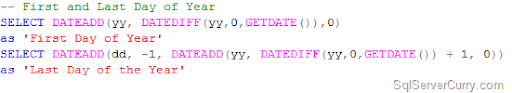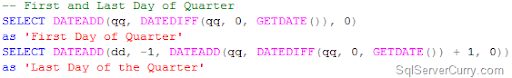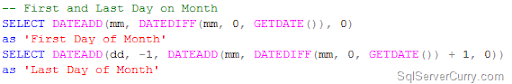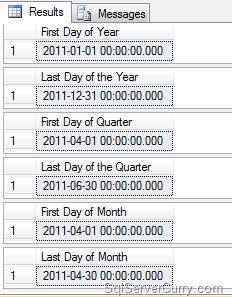### SQL Server: First and Last Day of Year, Quarter, Month and Week

With so many questions floating around calculating the first and last day of a given duration in SQL Server, here’s a post that explains the technique of calculating the first and last day of a given duration. We will cover the following:

- Calculate First and Last Day of the Year
- Calculate First and Last Day of the Quarter
- Calculate First and Last Day of a Month

First and Last Day of YearTo get the first day of the year, we are using the DATEDIFF function to determine the number of years from ‘1/1/1900’ to the current date (GETDATE). We then add this calculated number result (in this case 111) to ‘1/1/1900’, to obtain the first day of the year.

Similarly, a simple trick is adopted to get the last day of the year. We get the first date of the ‘next year’ and simply subtract one day from it. So the expression SELECT DATEADD(yy, DATEDIFF(yy,'',GETDATE()) + 1, 0) gets you the first day of the ‘next’ year. Subtracting 1 from it gives you the last day of the current year.
The same approach is adopted to find the last day of the quarter and the month.

First and Last Day of the QuarterFirst and Last Day of a MonthHere are all the three queries for you to try out:

-- First and Last Day of Year
as 'First Day of Year'
SELECT DATEADD(dd, -1, DATEADD(yy, DATEDIFF(yy,0,GETDATE()) + 1, 0)) as 'Last Day of the Year'

-- First and Last Day of Quarter
SELECT DATEADD(qq, DATEDIFF(qq, 0, GETDATE()), 0)
as 'First Day of Quarter'
as 'Last Day of the Quarter'

-- First and Last Day on Month
SELECT DATEADD(mm, DATEDIFF(mm, 0, GETDATE()), 0)
as 'First Day of Month'
as 'Last Day of Month'

OUTPUT